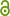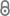# Controlling nonlinear PDEs using low-dimensional bilinear approximations obtained from data

S. Peitz, ArXiv:1801.06419 (2018).Preprint | English
Department
Abstract
In a recent article, we presented a framework to control nonlinear partial differential equations (PDEs) by means of Koopman operator based reduced models and concepts from switched systems. The main idea was to transform a control system into a set of autonomous systems for which the optimal switching sequence has to be computed. These individual systems can be approximated very efficiently by reduced order models obtained from data, and one can guarantee equality of the full and the reduced objective function under certain assumptions. In this article, we extend these results to continuous control inputs using convex combinations of multiple Koopman operators corresponding to constant controls, which results in a bilinear control system. Although equality of the objectives can be carried over when the PDE depends linearly on the control, we show that this approach is also valid in other scenarios using several flow control examples of varying complexity.
Publishing Year
Journal Title
arXiv:1801.06419
LibreCat-ID

### Cite this

Peitz S. Controlling nonlinear PDEs using low-dimensional bilinear approximations  obtained from data. arXiv:180106419. 2018.
Peitz, S. (2018). Controlling nonlinear PDEs using low-dimensional bilinear approximations  obtained from data. ArXiv:1801.06419.
@article{Peitz_2018, title={Controlling nonlinear PDEs using low-dimensional bilinear approximations  obtained from data}, journal={arXiv:1801.06419}, author={Peitz, Sebastian}, year={2018} }
Peitz, Sebastian. “Controlling Nonlinear PDEs Using Low-Dimensional Bilinear Approximations  Obtained from Data.” ArXiv:1801.06419, 2018.
S. Peitz, “Controlling nonlinear PDEs using low-dimensional bilinear approximations  obtained from data,” arXiv:1801.06419. 2018.
Peitz, Sebastian. “Controlling Nonlinear PDEs Using Low-Dimensional Bilinear Approximations  Obtained from Data.” ArXiv:1801.06419, 2018.Closed Access